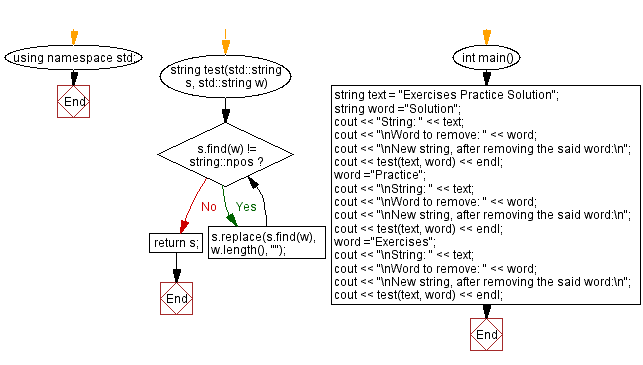﻿ C++ - Remove a word from a given string

# C++ String Exercises: Remove a word from a given string

## C++ String: Exercise-34 with Solution

Write a C++ program that removes a specific word from a given string. Return the updated string.

Test Data:
("Exercises Practice Solution", "Solution") -> "Exercises Practice"
("Exercises Practice Solution", "Practice ") -> "Exercises Solution"
("Exercises Practice Solution", " Solution") -> " Practice Solution"

Sample Solution:

C++ Code:

``````#include <bits/stdc++.h>

using namespace std;
string test(std::string s, std::string w)
{
while (s.find(w) != string::npos)
s.replace(s.find(w), w.length(), "");
return s;
}
int main()
{
string text = "Exercises Practice Solution";
string word ="Solution";
cout << "String: " << text;
cout << "\nWord to remove: " << word;
cout << "\nNew string, after removing the said word:\n";
cout << test(text, word) << endl;
word ="Practice";
cout << "\nString: " << text;
cout << "\nWord to remove: " << word;
cout << "\nNew string, after removing the said word:\n";
cout << test(text, word) << endl;
word ="Exercises";
cout << "\nString: " << text;
cout << "\nWord to remove: " << word;
cout << "\nNew string, after removing the said word:\n";
cout << test(text, word) << endl;
}
``````

Sample Output:

```String: Exercises Practice Solution
Word to remove: Solution
New string, after removing the said word:
Exercises Practice

String: Exercises Practice Solution
Word to remove: Practice
New string, after removing the said word:
Exercises  Solution

String: Exercises Practice Solution
Word to remove: Exercises
New string, after removing the said word:
Practice Solution
```

Flowchart:C++ Code Editor: